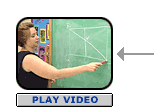# Polygons Part A: Hidden Polygons (20 minutes)

## Session 3, Part A

### In This Part

• Identifying Polygons
• Finding Polygons

### Identifying Polygons

Polygons are two-dimensional geometric figures with these characteristics:

• They are made of straight line segments.
• Each segment touches exactly two other segments, one at each of its endpoints.
• They are closed — they divide the plane into two distinct regions, one “inside” and the other “outside” the polygon.

These shapes are polygons:These shapes are not polygons:### Problem A1

Look at the shapes above that are not polygons. Explain why each of these shapes does not fit the definition of a polygon.

Polygons can be classified according to the number of sides they have. Note 2

 Name # of Sides Examples Triangle 3Quadrilateral 4Pentagon 5Hexagon 6Heptagon 7Octagon 8Nonagon 9Decagon 10Polygons with more than 10 sides are not usually given special names. A polygon with 11 sides is described as an 11-gon, a polygon with 12 sides as a 12-gon, and so on. Each of the polygons below is a 17-gon.When people talk about a general polygon — one where you don’t know the exact number of sides—they often refer to it as an n-gon.

Hidden Polygons and the Video Segment problem adapted from IMPACT Mathematics, developed by Educational Development Center, Inc. pp. 42-45. © 2000 Glencoe/McGraw-Hill. Used with permission. www.glencoe.com/sec/math

### Finding Polygons

Each corner of a polygon, where two sides meet, is called a vertex. The plural of vertex is vertices. Labeling vertices with capital letters makes it easy to refer to a polygon by name. For example, this figure contains two triangles and one quadrilateral:To name one of the polygons in the figure, list its vertices in order as you move around it in either direction. One name for the shaded triangle is Triangle ABC. Other names are possible, including BCA and ACB. One name for the white triangle is Triangle ADC.

The quadrilateral in the figure could be named Quadrilateral ABCD, BCDA, DCBA, or DABC. All of these names list the vertices in order as you move around the quadrilateral. The name ACBD is not correct.

In the following activities, you will search for polygons in several figures. You’ll calculate a score for each figure by adding the following:

1. 3 points for each triangle
2. 4 points for each quadrilateral
3. 5 points for each pentagon
4. 6 points for each hexagon

Be careful to give only one name for each polygon. You may want to record your work for each problem in a table like this one, which shows the result for this figure.As you work, try to discover a systematic way to find and list all the polygons in a figure.Polygon Names Score Triangle ABC, ADC 6 Quadrilateral ABCD 4 Pentagon None – Hexagon None – Total Score 10Tip: Here’s a sample strategy for counting: Count triangles and quadrilaterals, and then look for their “complements.” So YXWZV is everything in the shape except triangle YVZ. Another strategy would be to choose one vertex (e.g., X). Count all of the triangles that contain X. Then count all of the quadrilaterals that contain X, and so on. Next, count all of the triangles that contain vertex Y but not X, and so on.

### Problem A2

How many polygons can you find in the following figure?### Problem A3

How many polygons can you find in the following figure?### Problem A4

How many polygons can you find in the following figure?### Video Segment

In this video segment, Ric and Michelle discuss their strategies for finding polygons in various figures. Watch this segment after you have completed Problems A2-A4, and compare your strategies with those of the onscreen participants.

What kinds of strategies did Ric and Michelle use to find polygons in their figures? Did you use any other strategies?

You can find this segment on the session video approximately 6 minutes and 46 seconds after the Annenberg Media logo.

### Notes

Note 2

Discuss or reflect on why there is no name for a two-sided polygon. Namely, if two segments meet only at endpoints, the figure cannot be closed; therefore it cannot be a polygon.

### Problem A1

The first two shapes are not polygons because they are not made of straight line segments. The third shape is not a polygon because it is not closed, while the fourth shape divides the plane into three regions, rather than two.

### Problem A2

There are 13 polygons. They are as follows:

• Four small triangles, each defined by one side of the rectangle and two halves of the diagonals (e.g., XYV)
• Four pentagons, each a complement of one of the small triangles (e.g., VYZWX)
• Four large triangles, each defined by two sides of the rectangle and one of the diagonals (e.g., triangle XZW)
• The rectangle XYZW

Score: (8 • 3) + (1 • 4) + (4 • 5) = 48 points

### Problem A3

There are 13 polygons. They are as follows:

• Four small rectangles, all of which share the vertex Q (e.g., SMPQ)
• Four hexagons, each a complement of one of the small rectangles (e.g., PNOLSQ)
• Four larger rectangles, each defined by two small rectangles sharing one side (e.g., MNTS)
• The rectangle MNOL

Score: (9 • 4) + (4 • 6) = 60 points

### Problem A4

There are 13 polygons. They are as follows:

• Two small triangles (RUV and TWV)
• Their two complements (hexagons VUSTQR and VWQRST)
• Two quadrilaterals (RQWV and TSUV)
• Their two complements (pentagons VRSTW and VTQRU)
• Two larger triangles (RQT and TSR)
• Three rectangles (RUWQ, USTW, and QRST)

Score: (4 • 3) + (5 • 4) + (2 • 5) + (2 • 6) = 54 points